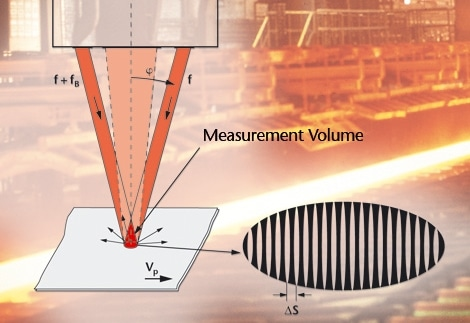# The Basic Principles of VelocimetryLaser Doppler Principle is used by Laser Surface Velocimeters (LSVs) to assess the laser light that is scattered back from a moving object. The LSVs from Polytec feature advanced detection circuits that are based on heterodyne detection to determine the light’s Doppler shift.

Polytec's heterodyne detection circuits, unlike conventional non-contact methods that only determine the magnitude of the velocity, can detect changes in direction, the direction of motion (+/-), as well as standstill conditions. The measurement is so precise that even small changes and displacements in velocity can be detected, thus enabling tighter process control.

## The Differential Doppler Process

Polytec Velocimeters work on the basis of the so-called Difference Doppler Technique. In this method, two coplanar laser beams are used to form a triangle with a vertex where the intersecting beams overlap on the surface being measured. This vertex is bisected by the instrument’s optical axis, which is coplanar with the laser beams making an angle φ with each beam. Further, the instrument is positioned in such a way that the plane is aligned with the surface velocity and perpendicular to the surface being measured.

The frequencies of the two laser beams are Doppler shifted for point P, which moves at velocity v through the intersection point of the two laser beams. The downstream beam is frequency shifted up and the upstream beam is frequency shifted down.

## Graphic Representation of the Difference Doppler Technique

The two laser beams are superimposed in the measurement volume and create an interference pattern of bright and dark fringes in this spatial area. The fringe spacing (Δs) is a system constant which is dependent on the laser wavelength (λ) and the angle between the laser beams (2φ):

Δs = λ/(2 sin φ)

When a particle moves via the fringe pattern, the intensity of the light it scatters back is modulated.

Due to this reason, an AC signal is generated by a photo receiver integrated in the sensor head. The frequency (fD) of this AC signal is directly proportional to the velocity component of the surface in measurement direction (vp) and it can be said that:

fD = vp/Δs = (2v/λ) sin φ

• Δs = Fringe spacing in the measurement volume
• vp = Velocity component in the direction of measurement
• fD = Doppler frequency

The λ/sinφ value makes up the material measure for the length and velocity measurement. After this value is precisely measured for every sensor head, it is printed on the identification label.This information has been sourced, reviewed and adapted from materials provided by Polytec.

## Citations

• APA

Polytec. (2019, October 17). The Basic Principles of Velocimetry. AZoM. Retrieved on October 20, 2020 from https://www.azom.com/article.aspx?ArticleID=13714.

• MLA

Polytec. "The Basic Principles of Velocimetry". AZoM. 20 October 2020. <https://www.azom.com/article.aspx?ArticleID=13714>.

• Chicago

Polytec. "The Basic Principles of Velocimetry". AZoM. https://www.azom.com/article.aspx?ArticleID=13714. (accessed October 20, 2020).

• Harvard

Polytec. 2019. The Basic Principles of Velocimetry. AZoM, viewed 20 October 2020, https://www.azom.com/article.aspx?ArticleID=13714.

## Tell Us What You Think